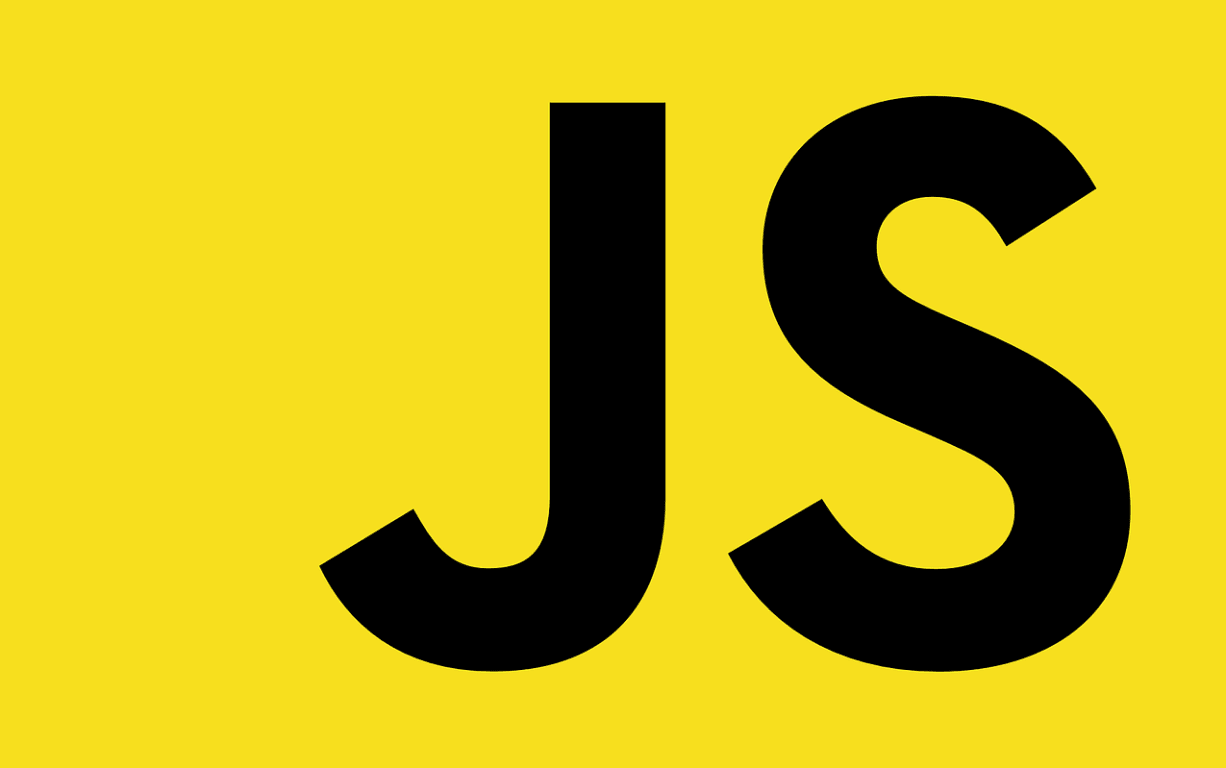# JavaScript Basics Part-2 (Variables)

Ed Tech
17 Jul 2020
Give a thumbs up
3

In this article, we explain some of the core features of the programming languages for giving you a better understanding of how JavaScript works. These features are common to all programming languages so now you just learn about these fundamentals.

You can try example code into your JavaScript console to see what output comes.

#### Variables

Variables are like containers that store values. There are three types of variables for storing values.

1. var (The values of This variable used globally).

2. let (This variable use as a local variable).

3. const (It contains constant values).

How do variables work?

Examples:

`var myVariable;`

Semicolons are used for at the end of a line indicates where a statement ends. This is only used when you need to separate statements on a single line.

You can choose your own variable name, but there are some restrictions.

• No spaces are allowed in a variables name
• You can use programming language cases (Camel case, Snake case, Kebab case, Pascal case, Upper case) for declaring a variable name.

JavaScript is a case sensitive programming language.

Example:

```var myVariable;
and
var myvariable;```

It means myVariable is not the same as myvariable.

When you declare a variable then you can give it a value:

```var myVariable;
myVariable = 'John';```

Also, you can give values on the same line:

`var myVariable = 'John';`

You can get values by calling the variable name:

`myVariable;`

If you want to change the given value, you can change it like the code given below:

```var myVariable = 'John';
myVariable = 'Smith';```

Variables hold different data types.

There are five common data types:

• String
• Number
• Boolean
• Array
• Object

1. String

The sequence of text is known as a string. A string, enclosed by a single quote mark.

Example:

`var myVariable = 'John';`

2. Number

It is used for storing a number. Numbers are not enclosed by quotes.

Example:

`var myVariable = 50;`

3. Boolean

Boolean only stores True/False value. Words true and false are special keywords so don't need quote marks.

Example:

```var myVariable1 = true;
var myVariable2 = false;```

4. Array

It is used for storing multiple values in a single line reference.

```var myVariable = [50, 'John', 'Smith', 20];

Get values to each member of the array like this:

myVariable, myVariable, etc.

Array's indexing start from 0.```

5. Object

It's used for anything. Store everything in a variable to a JavaScript object.

```var myVariable = document.querySelector('h1');

Like the above examples.```

Variables are required to do anything in programming.